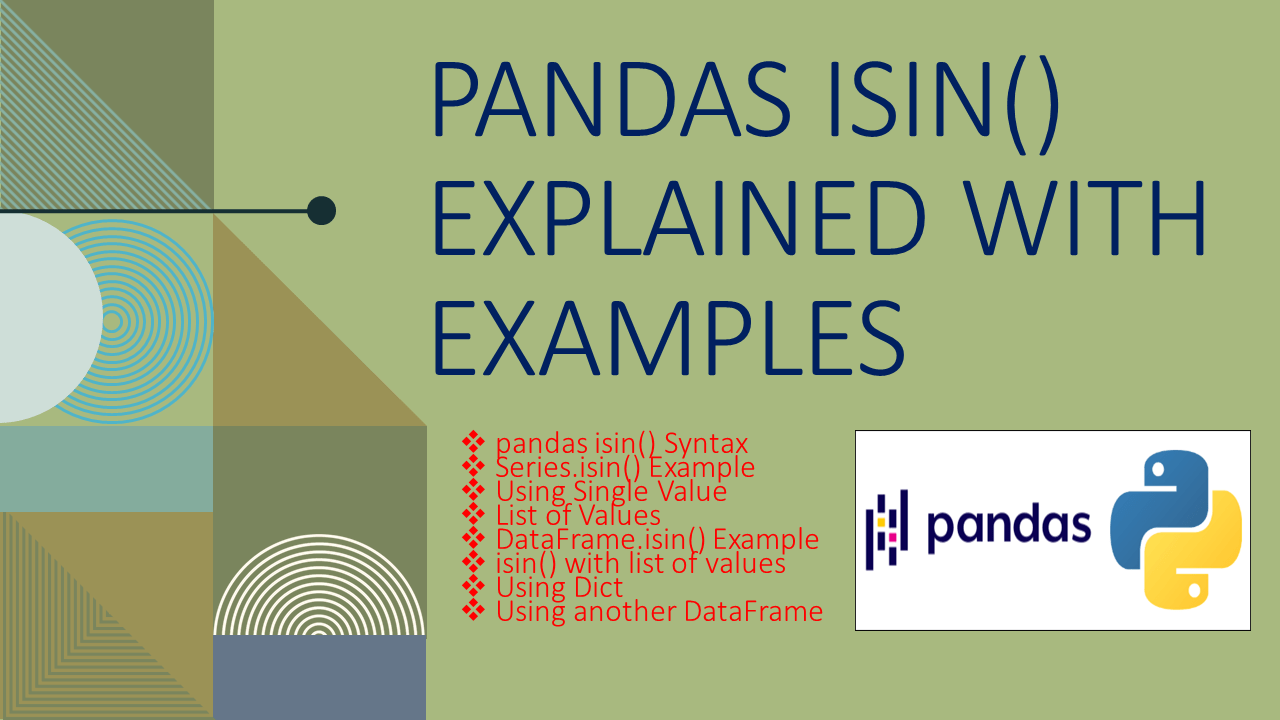# pandas isin() Explained with Examples

• Post author:
• Post category:Pandas

Pandas isin() function exists in both DataFrame & Series which is used to check if the object contains the elements from list, Series, Dict. It returns the same as the caller object of booleans indicating if each row cell/element is in values.

When it is called on DataFrame it returns a Pandas DataFrame with a boolean value representing whether it has a value or not. When it is called on Pandas Series, it returns a Series of booleans indicating if each element is in values.

pandas isin() Key Points –

• isin() function exists in both DataFrame and Series.
• It returns the same object as the caller with boolean values.
• Represented as True when value present, otherwise False.
• By using Series.isin(), you can filter the DataFrame.

## 1. pandas isin() Syntax

Following is the syntax of the isin() function. This takes any thing from iterable, Series, and Dict values as parameters.

``````
# Syntax of isin() Function
isin(values)
``````

Values parameter accept the following.

• `values`iterable, Series, DataFrame or dict

Let’s create a DataFrame and learn how to use isin() function with examples.

``````
# Create a pandas DataFrame.
import pandas as pd
df = pd.DataFrame({
'Courses' :['Spark','Python','Java'],
'Fee' :[22000,25000,23000,],
'Duration':['30days','50days','30days']
})
print(df)

# Output:
#  Courses    Fee Duration
# 0   Spark  22000   30days
# 1  Python  25000   50days
# 2     Java  23000   30days
``````

## 2. Series.isin() Example

pandas Series.isin() function is used to filter the DataFrame rows that contain a list of values. When it is called on Series, it returns a Series of booleans indicating if each element is in values, `True` when present, `False` when not. You can pass this series to the DataFrame to filter the rows.

### 2.1. Using Single Value

The below example checks each element of the `Courses` column contains the value `Spark`, when present it represents `True`. Returns rows that have `Spark` in column `Courses`.

``````
# Specific Value
df2=df[df['Courses'].isin(['Spark'])]
print(df2)

# Output:
#  Courses    Fee Duration
# 0   Spark  22000   30days
``````

### 2.1. isin() with List of Values

This checks if the column `Courses` contains in the list of values by using Pandas isin(). It returns rows where `Courses` contains `Spark`, `Java`.

``````
# List of Values
df2=df[df['Courses'].isin(['Spark','Java'])]
print(df2)

# Output:
#  Courses    Fee Duration
# 0   Spark  22000   30days
# 2    Java  23000   30days
``````

## 3. DataFrame.isin() Example

Below are examples of how to use DataFrame.isin() function.

### 3.1 isin() with list of values

When a python list is passed as a parameter value to the Pandas DataFrame.isin() function, it checks whether each cell value from DataFrame is present in the list, if found, shows `True` otherwise `False` (When a value is not present). The resultant DataFrame just contains boolean values.

``````
# isin() with list of values
print(df.isin(['Spark','Python',23000,'50days']))

# Output:
#   Courses    Fee  Duration
# 0     True  False     False
# 1     True  False      True
# 2    False   True     False
``````

### 3.2 Using Dict

The above example doesn’t check values in a specific DataFrame column, In order to check the values in a specific column use the Python Dictionary object as param. When a python Dict is passed as a param to the isin(), you should have a column name as the key and elements you wanted to check as Dict value. With this, you can check values in multiple columns.

``````
# check by column name
print(df.isin({'Courses': ['Spark', 'Python']}))

# Output:
#   Courses    Fee  Duration
# 0     True  False     False
# 1     True  False     False
# 2    False  False     False
``````

### 3.3 Using another DataFrame

You can also check with another DataFrame.

``````
# Checks in another DataFrame
df2 = pd.DataFrame({
'Courses' :['C++','Python',],
'Fee' :[23000,25000,],
'Duration':['30days','55days']
})
print(df.isin(df2))

# Output:
#   Courses    Fee  Duration
# 0    False  False      True
# 1     True   True     False
# 2    False  False     False
``````

## 4. Complete Example of DataFrame & Series isin()

``````
# Create a pandas DataFrame.
import pandas as pd
import numpy as np

df = pd.DataFrame({
'Courses' :['Spark','Python','Java'],
'Fee' :[22000,25000,23000,],
'Duration':['30days','50days','30days']
})
print(df)

# List of values
print(df.isin(['Spark','Python',23000,'50days']))

# Check by column
print(df.isin({'Courses': ['Spark', 'Python',23000]}))

# Using DataFrame
df2 = pd.DataFrame({
'Courses' :['C++','Python',],
'Fee' :[23000,25000,],
'Duration':['30days','55days']
})
print(df.isin(df2))

# Single value
df2=df[df['Courses'].isin(['Spark'])]
print(df2)

# Multiple values
df2=df[df['Courses'].isin(['Spark','Java'])]
print(df2)
``````

## 5. Conclusion

In this article, you have learned isin() function exists in both DataFrame & Series which is used to check if the object contains the elements from a list, Series, and Dict.

## References

### Naveen

I am a Data Engineer with 20+ years of experience in transforming data into actionable insights. Over the years, I have honed my expertise in designing, implementing, and maintaining data pipelines with frameworks like Apache Spark, PySpark, Pandas, R, Hive and Machine Learning. My journey in the field of data engineering has been a continuous learning, innovation, and a strong commitment to data integrity. I have started this SparkByExamples.com to share my experiences with the data as I come across. You can learn more about me at LinkedIn